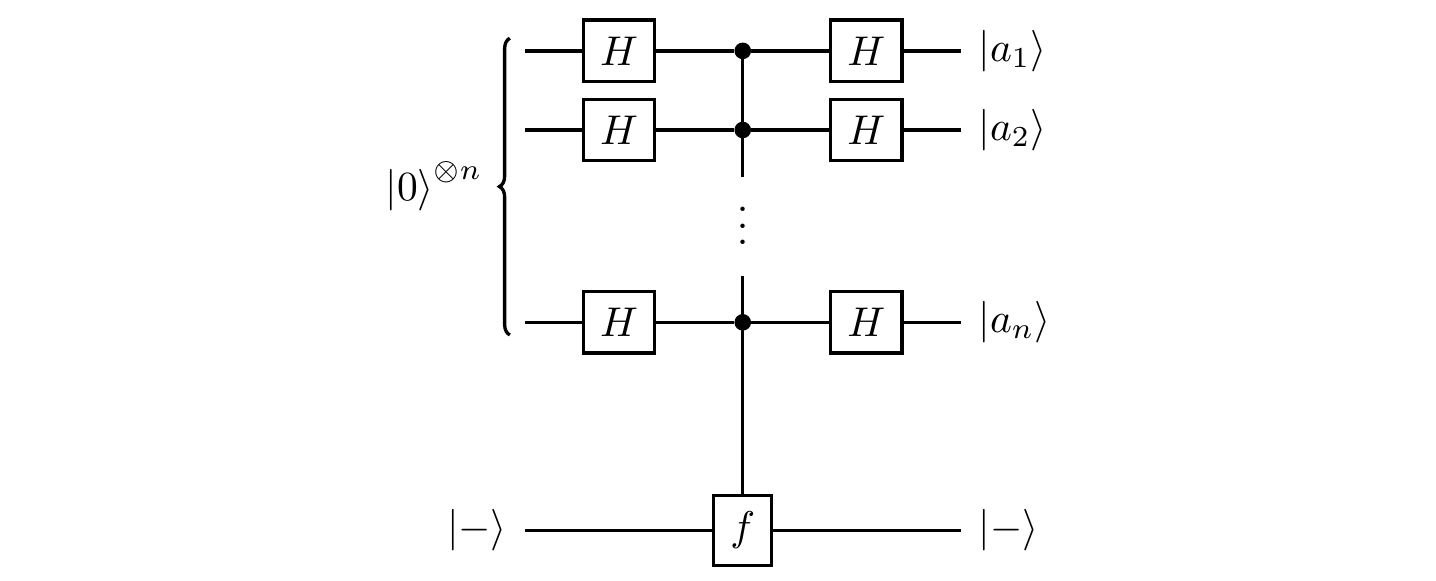## 10.5 The Bernstein–Vazirani algorithm

(Hidden inner-product determination).

We are presented with an oracle that computes some unknown function f\colon\{0,1\}^n\to\{0,1\}, but we are promised that f is of the form f(x) = a\cdot x \equiv (a_1\cdot x_1) \oplus \ldots \oplus (a_n\cdot x_n) for some fixed binary string a=a_1a_2\ldots a_n\in\{0,1\}^n.

Our task is to determine, using the fewest queries possible, the value of the n-bit string a.

It’s quite easy to see how to do this classically: if we input the value x=00\ldots010\ldots0, where the m-th bit is a 1 and all other bits are 0, then f(x) is simply the m-th bit of a; after n such calls, we will know every bit value, and thus know a. It is also clear that there cannot exist a better classical algorithm: each call to the oracle teaches us exactly one bit of information, and since we must learn n bits, we must query it n times.

In contrast, by running the circuit below, it is possible to determine the value of a with a single call to the oracle!201

(The Bernstein-Vazirani algorithm).

First register: n qubits. Second register: 1 qubit.A quick note on notation: the “\ldots” in the circuit means “there are more wires here but they are identical (apart from the numbering) to the ones above”. You might also see other notation to denote this, such asor even simplyNow, stepping through the execution of the circuit (and ignoring the second register, which, as per usual, remains in the state |-\rangle throughout), we obtain \begin{aligned} |0^{\otimes n}\rangle &\overset{H^{\otimes n}}{\longmapsto} \left(\frac{1}{\sqrt{2}}\right)^n \sum_{x\in\{0,1\}^n} |x\rangle \\&\overset{U_f}{\longmapsto} \left(\frac{1}{\sqrt{2}}\right)^n \sum_{x\in\{0,1\}^n} (-1)^{a\cdot x}|x\rangle \\&\overset{H^{\otimes n}}{\longmapsto} \left(\frac{1}{\sqrt{2}}\right)^n \sum_{x\in\{0,1\}^n} \left[ (-1)^{a\cdot x} \left(\frac{1}{\sqrt{2}}\right)^n \sum_{y\in\{0,1\}^n} (-1)^{y\cdot x} |y\rangle \right] \\&\quad= \left(\frac{1}{2}\right)^n \sum_{y\in\{0,1\}^n} \left[ \sum_{x\in\{0,1\}^n} (-1)^{(a\oplus y)\cdot x} \right] |y\rangle \end{aligned} where we write the second Hadamard transform as |x\rangle \longmapsto \left(\frac{1}{\sqrt{2}}\right)^n \sum_{y\in\{0,1\}^n} (-1)^{y\cdot x}|y\rangle.

To see that this output is indeed equal to |a\rangle, we can use the fact202 that, for any y\in\{0,1\}^n, \sum_{x\in\{0,1\}^n} (-1)^{x\cdot y} = \begin{cases} 0 &\text{if $y\neq0$;} \\2^n &\text{if $y=0$} \end{cases} which, in our case, tells us that \sum_{x\in\{0,1\}^n} (-1)^{(a\oplus y)\cdot x} = \begin{cases} 0 &\text{if $y\neq a$;} \\2^n &\text{if $y=a$.} \end{cases}

In other words, if you take the sum over x, then all the terms always cancel out unless a\oplus y = 00\ldots0, but this happens if and only if y=a. Then the standard bit-by-bit measurement of the first register gives the value of a and solves the problem with a single call to the oracle.

Alternatively, if you don’t immediately see how this sum works for z\neq a (where we write |z\rangle to mean the output), you can first calculate the probability that the output is z=a. In this case it is easy to see that the sum is 2^n, and that in the final state \sum_z\lambda_z|z\rangle the term z=a has amplitude 1. Thus, by normalisation, all the other terms must be equal to 0.

1. This algorithm is named for Ethan Bernstein and Umesh Vazirani who proposed it in 1997.↩︎

2. Exercise 10.12.5.↩︎